# CUDA 共享内存 bank conflict

### 1. bank conflict

GPU 共享内存是基于存储体切换的架构（bank-switched-architecture）。在 Femi，Kepler，Maxwell 架构的设备上有 32 个存储体（也就是常说的共享内存分成 32 个bank），而在 G200 与 G80 的硬件上只有 16 个存储体。

__shared__ float sData;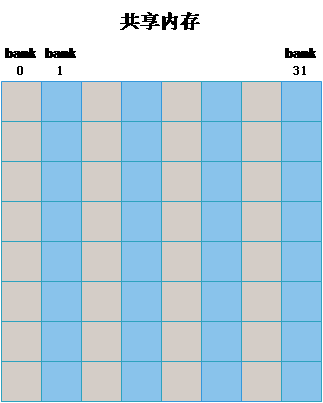1. 同常量内存一样，当一个 warp 中的所有线程访问同一地址的共享内存时，会触发一个广播（broadcast）机制到 warp 中所有线程，这是最高效的。
2. 如果同一个 warp 中的线程访问同一个 bank 中的不同地址时将发生 bank conflict。
3. 每个 bank 除了能广播（broadcast）还可以多播（mutilcast）（计算能力 >= 2.0），也就是说，如果一个 warp 中的多个线程访问同一个 bank 的同一个地址时（其他线程也没有访问同一个bank 的不同地址）不会发生 bank conflict。
4. 即使同一个 warp 中的线程 随机的访问不同的 bank，只要没有访问同一个 bank 的不同地址就不会发生 bank conflict。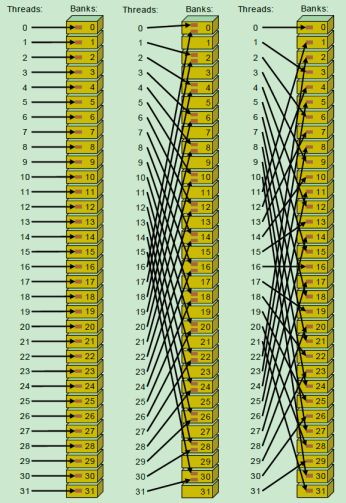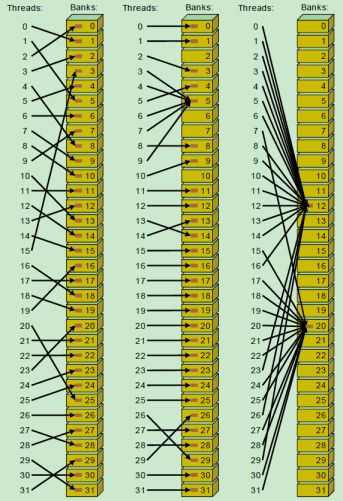### 2. 实验 1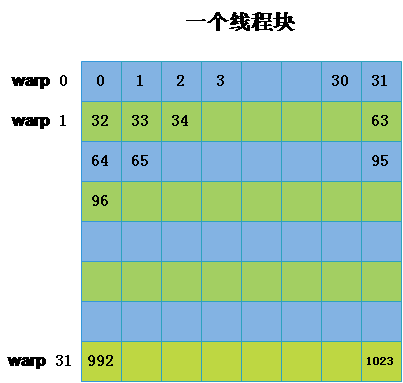• 实验 1.1
该线程块中的每个 warp 读写不同的 bank，不同的 warp 不会访问一个地址，也就是一一对应的关系。图中的数字就表示上图中的线程标号。经分析可知，此时是没有 bank conflict 的。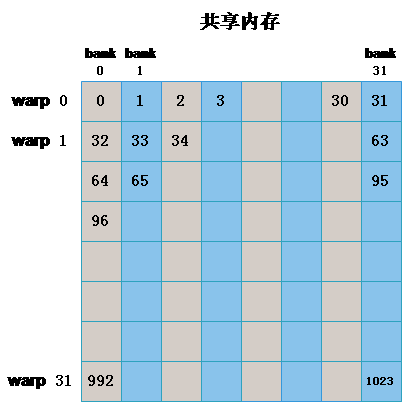int x_id = blockDim.x * blockIdx.x + threadIdx.x; // 列坐标
int y_id = blockDim.y * blockIdx.y + threadIdx.y; // 行坐标
int index = y_id * col + x_id;

__shared__ float sData[BLOCKSIZE][BLOCKSIZE];

if (x_id < col && y_id < row)
{
sData[threadIdx.y][threadIdx.x] = matrix[index];
__syncthreads();
matrixTest[index] = sData[threadIdx.y][threadIdx.x];
}
• 实验 1.2
该线程块中的每个 warp 读写相同的 bank 的不同地址，不同的 warp 访问不同，也就是一一对应的关系。图中的数字就表示上图中的线程标号。经分析可知，此时是存在很严重的 bank conflict 。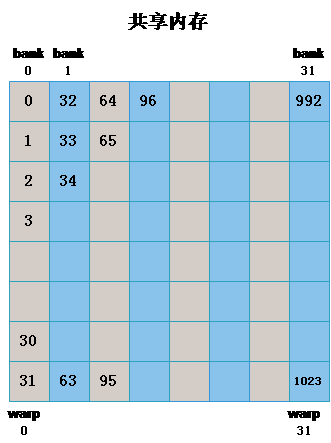int x_id = blockDim.x * blockIdx.x + threadIdx.x; // 列坐标
int y_id = blockDim.y * blockIdx.y + threadIdx.y; // 行坐标
int index = y_id * col + x_id;

__shared__ float sData[BLOCKSIZE][BLOCKSIZE];

if (x_id < col && y_id < row)
{
sData[threadIdx.x][threadIdx.y] = matrix[index];
__syncthreads();
matrixTest[index] = sData[threadIdx.x][threadIdx.y];
}
• 实验 1.3（避免 bank conflict 的技巧）
针对实验 1.2 中出现的严重的 bank conflict，我们可以通过添加一个附加列来避免 bank conflict，如下图所示，左图为申请的共享内存矩阵形式，右图是表示成 bank 后的形式，通过这种方式，原来在一个 bank 中的同一个 warp 都正好偏移到了不同的 bank 中。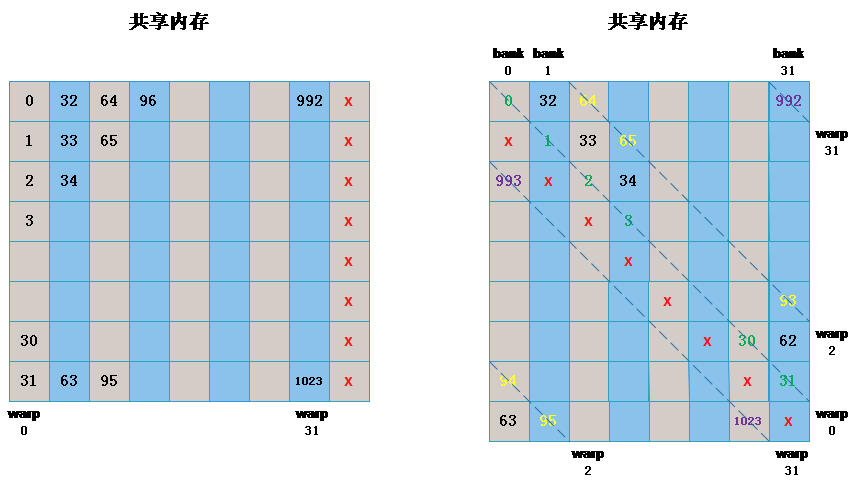int x_id = blockDim.x * blockIdx.x + threadIdx.x; // 列坐标
int y_id = blockDim.y * blockIdx.y + threadIdx.y; // 行坐标
int index = y_id * col + x_id;

__shared__ float sData[BLOCKSIZE][BLOCKSIZE+1];

if (x_id < col && y_id < row)
{
sData[threadIdx.x][threadIdx.y] = matrix[index];
__syncthreads();
matrixTest[index] = sData[threadIdx.x][threadIdx.y];
}

实验 1.1 ：0.052416 ms

实验 1.1 ：0.034816 ms

1. 通过额外的一行，可以避免 bank conflict，运行时间与完全没有 bank conflict 的运行时间差距很小。
2. 存在 bank conflict 的，运行时间几乎是没有 bank conflict 的运行时间的 4 倍。

### 3. 实验 2

• 实验 2.1
同一个 block 中所有第 i 列的线程都计算第 i 行的元素的和，此时所有同一个warp 会访问同一个 bank 的不同地址。如下图所示，分别表示第 0 列访问 bank 0 中的第一个地址，第 1 列访问 bank 1 中的第 1 个地址，依次类推。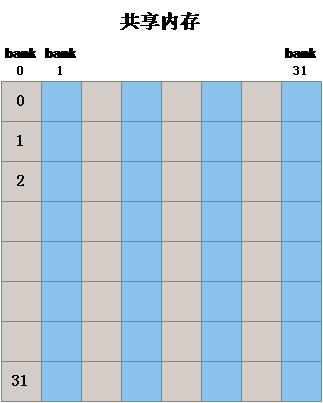int x_id = blockDim.x * blockIdx.x + threadIdx.x; // 列坐标
int y_id = blockDim.y * blockIdx.y + threadIdx.y; // 行坐标
int index = y_id * col + x_id;

__shared__ float sData[BLOCKSIZE][BLOCKSIZE];

if (x_id < col && y_id < row)
{
sData[threadIdx.y][threadIdx.x] = matrix[index];
__syncthreads();

float data = 0.0f;
for (int j = 0; j < BLOCKSIZE; j++)
{
data += sData[threadIdx.x][j];
}
matrixTest[index] = data;
}
• 实验 2.2
同实验 1.3 类似，添加额外的一列，如下图所示：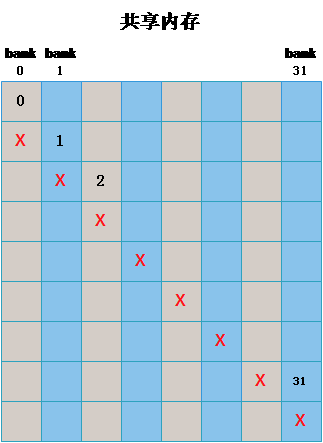int x_id = blockDim.x * blockIdx.x + threadIdx.x; // 列坐标
int y_id = blockDim.y * blockIdx.y + threadIdx.y; // 行坐标
int index = y_id * col + x_id;

__shared__ float sData[BLOCKSIZE][BLOCKSIZE+1];

if (x_id < col && y_id < row)
{
sData[threadIdx.y][threadIdx.x] = matrix[index];
__syncthreads();

float data = 0.0f;

for (int j = 0; j < BLOCKSIZE; j++)
{
data += sData[threadIdx.x][j];
}
matrixTest[index] = data;
}

实验 2.1 ：0.458144 ms

### 4. 实验 3

• 实验 3.1
采用实验 1.1 的方式，同一个 warp 访问不同的 bank，不同的 warp 访问不同的地址。

int x_id = blockDim.x * blockIdx.x + threadIdx.x; // 列坐标
int y_id = blockDim.y * blockIdx.y + threadIdx.y; // 行坐标
int index = y_id * col + x_id;

__shared__ float sData[BLOCKSIZE][BLOCKSIZE];

if (x_id < col && y_id < row)
{
sData[threadIdx.y][threadIdx.x] = matrix[index];
__syncthreads();

float data = 0.0f;
for (int j = 0; j < 1000; j++)
{
data = sData[threadIdx.y][threadIdx.x];
}
matrixTest[index] = data;
}
• 实验 3.2
同一个 warp 访问不同的 bank，所有 warp 访问同一个地址，也就是说所有的行都会访问第 0 行。

int x_id = blockDim.x * blockIdx.x + threadIdx.x; // 列坐标
int y_id = blockDim.y * blockIdx.y + threadIdx.y; // 行坐标
int index = y_id * col + x_id;

__shared__ float sData[BLOCKSIZE][BLOCKSIZE];

if (x_id < col && y_id < row)
{
sData[threadIdx.y][threadIdx.x] = matrix[index];
__syncthreads();

float data = 0.0f;
for (int j = 0; j < 1000; j++)
{
data = sData[threadIdx.x];
}
matrixTest[index] = data;
}

实验 2.1 ：0.053800 ms